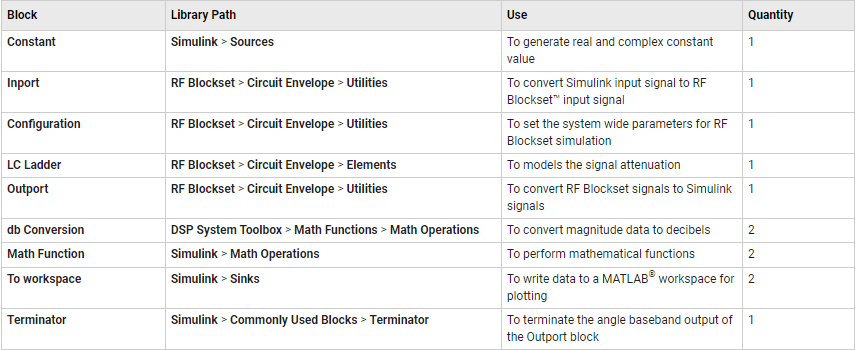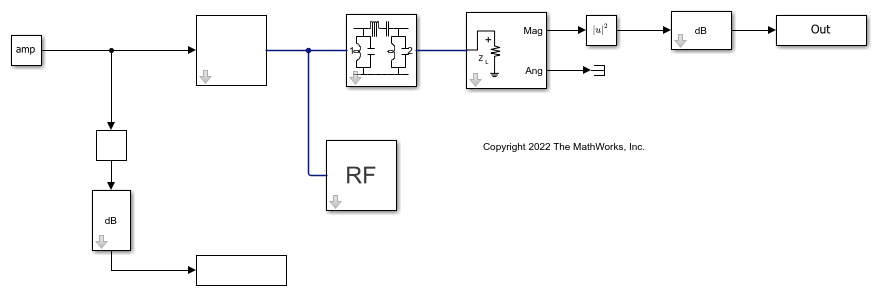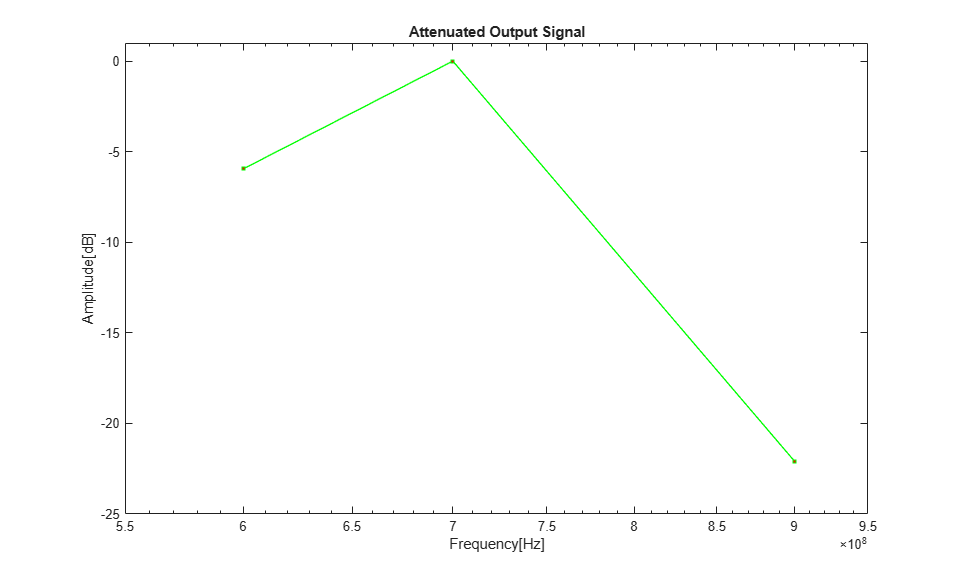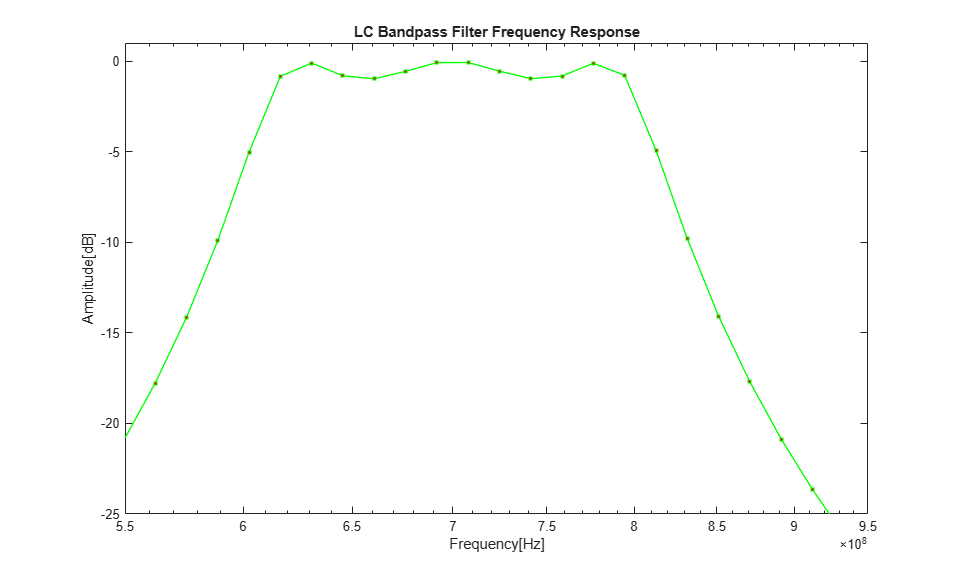# Model RF Filter Using Circuit Envelope

This example shows how to model an RF filter using Circuit Envelope Library. In this example, you compare the input and output signal amplitudes to study the signal attenuation.

### Model Overview

This example uses an LC bandpass filter designed to have a bandwidth of 200 MHz. The filter uses a three tone input signal to demonstrate the filter attenuation property for in-band and out-band frequencies. The input signal tones are:

• 700 MHz – Center frequency of the filter passband

• 600 MHz – Lower edge frequency of the filter passband

• 900 MHz – Frequency outside the filter passband

### Define Model Variables and Settings

Define model variables for blocks that share parameter values using the `InitFcn`.

• In Simulink® editor, select MODELING > SETUP > Model Settings > `Model Properties` .

• In the `Model Properties` dialog box, on the Callbacks tab in Model callbacks pane, select `InitFcn` .

• In the Model initialization function pane, enter: `amp = ones(1,3); freq = [600 700 900]*1e6; stepsize = 1/500e6;`

• Click OK.

• In the Simulink tool bar, set the simulation stop time to `0`.

• In Simulink editor, select MODELING > SETUP > Model Settings > `Model Settings Ctrl+E`

• In Configuration Parameters dialog box, set Solver to `discrete (no continuous states)` and in Data Import/Export pane, clear `Single simulation output`.

### Required Blocks

The filter system consists of LC Ladder, Inport, Outport and Configuration blocks. The physical part of the model uses bidirectional RF signals. The blocks used in the system are:Connect the blocks as shown in the model or open the model attached in this example.

```open_system("rffilter_ce.slx") ```### Configure Input Signal

Generate the three-tone input signal using these blocks:

• Constant block specifies the amplitude of the signal.

• Inport block configures the frequencies of the three tones.

• Configuration block specifies the step size.

`In the Constant block:`
• Set the Constant value to `amp`, as defined in the `InitFcn`.

In the Inport block:

• Set Source type to `Power`.

• Set Carrier frequencies to `freq` , as defined in `InitFcn`. The `freq` variable sets the frequency of the three tones to 600 MHz, 700 MHz, and 900 MHz, respectively.

• Click OK.

In the Configuration block:

• Set Step size to `stepsize`, as defined in `InitFcn`.

• Clear Simulate noise.

• Click OK.

The fundamental tones and harmonics are updated automatically when you run the model.

### Configure RF Filter

In the LC Ladder block:

• Set Ladder topology to `LC Bandpass Pi`.

• Click Apply and then click OK.

### Configure Output Settings

In the Outport block :

• Set Sensor type to `Power`.

• Set Output to `Magnitude and Angle Baseband`.

• Set Carrier frequencies to `freq`, as defined in `InitFcn`.

• Click OK.

### Configure Other Blocks

• In the Simulink editor, connect the `Ang` port of the Outport block to Terminator block to terminate the angle baseband output.

• In Math Function and Math Function 1 blocks, set Function to `magnitude^2` and click OK. The block squares the magnitude of the input and output signal.

• In dB Conversion and dB Conversion 1 blocks, set Input Signal to `Power` and click OK. The block converts the input and output signals to dB.

• In To Workspace, change the Variable name to `In`. In To Workspace 1, change the Variable name to `Out`. In both blocks, set Save format to Array and click OK.

### Run Model

Select Simulation > Run to run the model.

```sim("rffilter_ce.slx") ```

### Plot and Analyze Attenuated Output Signals

Display the input and output signals using `semilogx` function, in dB.

• Transfer the input and output dB values to the MATLAB® workspace using To Workspace block.

• To view the input signal to RF Filter, plot `In` array from the MATLAB workspace.

```figure h = semilogx(freq, In,'-gs','LineWidth',1,'MarkerSize',3,'MarkerFaceColor','r'); xlim([5.5e8,9.5e8]); xlabel('Frequency[Hz]'); ylabel('Amplitude[dB]'); title('Input Signal'); ```To view the output signal (filtered attenuated signal), plot `Out` array from the MATLAB workspace.

```figure h1 = semilogx(freq, Out,'-gs','LineWidth',1,'MarkerSize',3,'MarkerFaceColor','r'); xlim([5.5e8,9.5e8]); ylim([-25,1]); xlabel('Frequency[Hz]'); ylabel('Amplitude[dB]'); title('Attenuated Output Signal'); ```Compare the input and output signal plots to verify the attenuation caused by the filter. Notice that the RF filter does not attenuate the signal at the center frequency of 700 MHz.

### Analyze LC Bandpass Filter Response

Plot more points to better understand the response of the LC bandpass filter. Change the defined variables in Model Properties to: `amp = ones(1,201); freq = logspace (8,10,201); stepsize = 1/500e6;`.

Run the model. Notice that the signal is not attenuated within the 200 MHz range of the LC bandpass filter. Alternatively, you can run `rffilter_ce3.slx`. Note that `rffilter_ce3.slx` is loaded with the updated defined variables.

```sim("rffilter_ce3.slx") ```

Plot the LC bandpass filter frequency response.

```figure h3 = semilogx(freq, Out,'-gs','LineWidth',1,'MarkerSize',3,'MarkerFaceColor','r'); xlim([5.5e8,9.5e8]); ylim([-25,1]); xlabel('Frequency[Hz]'); ylabel('Amplitude[dB]'); title('LC Bandpass Filter Frequency Response'); ```